PPWIZARD is a free preprocessor for HTML, REXX, Visual Basic or any text files.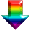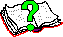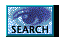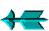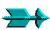\ -> Rexx -> Rexx Conditional Logic

 Rexx Conditional Logic

You can get a rexx manuals (for regina) from http://www.lightlink.com/hessling/, there are HTML and PDF versions available.

The #if command allows you to evaluate any rexx conditional expression to determine whether its true or not.

The following tests operators are concidered to be "non-strict" (leading and trailing blanks are ignored as are leading zeros on numbers):

• =
Tests if values are equal.

• <>
Tests if values are not equal.

• <
Tests if left value is less than the right value (can be text or number).

• <=
Tests if left value is less than or equal to the right value (can be text or number).

• >
Tests if left value is greater than the right value (can be text or number).

• >=
Tests if left value is greater than or equal to the right value (can be text or number).

The following tests operators are concidered to be "strict":

• ==
Tests if values are equal.

• \==
Tests if values are not equal.

• <<
Tests if left value is less than the right value (can be text or number).

• <<=
Tests if left value is less than or equal to the right value (can be text or number).

• >>
Tests if left value is greater than the right value (can be text or number).

• >>=
Tests if left value is greater than or equal to the right value (can be text or number).

The following logical operators are also useful:

• & - AND (both must be true)

• | - OR (either can be true)

• \ - NOT (reverses true/false)

Some examples follow:

```#if  lines(<\$LanguageFile>) = 0
#if  Defined('Var1') = 'Y' | Defined('Var2') = 'Y'
#if <\$TmpHH> < 12
#if translate(GetEnv("PRJSRCDIR")) = "E:\DB\PROJECTS\HOMEPAGE"
```

Saturday May 28 2022 at 2:55pm